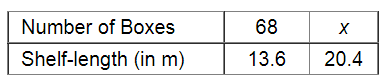# 68 boxes of a certain commodity require a shelf-length of 13.6 m.`
Question:

68 boxes of a certain commodity require a shelf-length of 13.6 m. How many boxes of the same commodity would occupy a shelf length of 20.4 m?

Solution:Let $x$ be the number of boxes that occupy a shelf-length of $20.4 \mathrm{~m}$.

If the length of the shelf increases, the number of boxes will also increase.

Therefore, it is a case of direct variation.

$\frac{68}{x}=\frac{13.6}{20.4}$

$\Rightarrow 68 \times 20.4=x \times 13.6$

$\Rightarrow x=\frac{68 \times 20.4}{13.6}$

$=\frac{1387.2}{13.6}$

$=102$

Thus, 102 boxes will occupy a shelf - length of $20.4 \mathrm{~m}$.